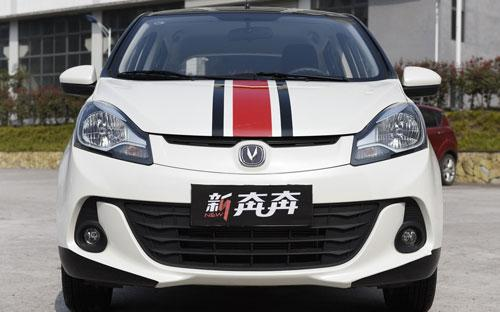### 长安 奔奔6 种颜色可选2015款最低售价：4.49 万元起

3730(mm)1650(mm)1530(mm)##### 配置亮点：
• 胎压监测装置

• ISOFIX儿童座椅接口

• 车身稳定控制(ESC/ESP/DSC等)

• 电动天窗

• 定速巡航

• 后倒车雷达

• 真皮座椅

• GPS导航系统

• 氙气大灯

• 后视镜加热

• 提交

长安 奔奔 绕车实拍• 长安 奔奔 在售车型

排量 车型 厂商指导价 本地最低报价 购车工具
1.4L
4.49万
4.49万

4.79万
4.79万

5.19万
5.19万

5.49万
5.49万

5.29万
5.69万
5.69万

5.99万
5.99万

4.59万
4.89万
4.89万

5.29万
5.29万

5.59万
5.39万
5.39万

5.79万
5.79万

6.09万

### 长安 奔奔 动力加速

奔奔 0-100公里加速时间分布在 0.0-秒 属于 超跑级

动力级别 加速时间 车型

长安 奔奔 视频

长安 奔奔 新闻资讯

# 早春二月 跟着新奔奔动起来吧

专业导购 超过8287次关注

二月，天气渐暖，万物复苏，是个出去踏青的好日子，作为有梦想、有情怀、标新立异、追求自由的90后，什么样的爱车才是今春踏青出游最IN的标配呢？长安新奔奔，一辆...

# 动力澎湃科技有范 新奔奔实力爆棚

专业导购 超过7483次关注

长安汽车全球战略性精品小车，成为在小型车市场对抗合资车型的主力之一。新奔奔不仅造型时尚前卫，更集合前沿的配置和澎湃的动力等优势于一身。同时对细节把控更是...

# 长安新奔奔强势逆袭 领跑小型车市场

国产新车 超过10441次关注

2015年的市场遭遇增幅放缓，但依然有长安奔奔系列这样的小型车独领风骚，成为国内小型车市场的领头羊车型。

# 新奔奔潮范儿演绎时尚魅力

新闻 超过7707次关注

随着市场发展和年轻群体消费需求的变化，彰显个性的双色车身设计越来越深受欢迎。继3月份推出的新奔奔主题PPO车型后，于8月份，新奔奔紧跟潮流发展，再次新增四款双...

# 精品新奔奔小块头有大品质

国产新车 超过9001次关注

作为长安旗下精品小车的新奔奔不仅凭借时尚造型、炫酷科技和澎湃动力等优势卖点做到领先同级，而今年3月份的全面转产铃木，则充分证明了新奔奔的品质已达到合资标准。

# 新奔奔炫酷配置专为新生代

国产新车 超过9150次关注

年轻的85后、90后逐渐成为汽车市场的主流消费群体，定位为视觉系乐趣小车的新奔奔充分研究年轻人的消费需求，比如搭配全景天窗，还有方向盘拨片换挡等多项同级领先...

# 新奔奔升级版PPO 潮范现身

国产新车 超过10786次关注

8月28日，长安汽车新奔奔升级版PPO车型在天猫旗舰店正式上市，9月份将陆续到店发售。共推出赤焰战将、果宝特工、赛道精灵（TS版）和逐风侠（TS版）四种版本车型。

# 长安新奔奔演绎年轻无极限

国产新车 超过7351次关注

定位为“视觉系乐趣小车”的新奔奔，继今年3月份推出三款主题PPO车型后，将再次于9月推出四款升级版PPO车型，搭配双色车身和双色内饰，为时尚潮群带来全新的购车体验。

# 实拍奔奔1.4L手动豪华型 解读最低配车型

评测 超过4765次关注

作为长安汽车在2014年的重点投放车型，全新一代奔奔有很多吸引人之处，比如全景天窗、旋钮换挡、换挡拨片等等。当然，这些配置肯定不会覆盖整个车系的，比如本文即...

# 后生可畏实力不浅 试驾长安新奔奔1.4L

试驾评测 超过6785次关注

随着汽车的普及,拥堵的交通、找停车位难开始成为每一位司机头疼的事，微型车或许能够解决一些不必要的麻烦。提起微型车我们脑海中大部分都会联想到奇瑞QQ、奥拓、比...

# 启辰R30竞争力分析 长安汽车新奔奔

导购 超过4281次关注

​长安奔奔是一款自主品牌车型，定位为微型车。老款奔奔也曾凭借着实惠的售价拥有不错的销量。经过了三年多的时间打造出的全新奔奔，可谓是发生了颠覆性的改变，更...

# 奔奔自动挡即将上市 消费者问题解析

导购 超过3535次关注

等待新车也好，审视老款车性价出手也罢，我们都希望作为消费者的您能够找到一款最适合的车，能够对它有所了解，提高对您爱车的认知度，减少疑惑。您如果有问题，可...

# 长安新奔奔即将上市 预计4万元起售

新闻 超过3308次关注

近日，据相关人士透露，长安新奔奔即将正式上市。预计，该款换代新车将会搭载一款1.4L发动机，售价区间为4万-6万元，该款新车的竞争对手将会锁定奇瑞QQ、奥拓、比亚...

# 新奔奔将于明年一季度上市 预计4万元起

新闻 超过3034次关注

近日，小编获悉，长安新一代奔奔或将于明年一季度正式上市。新奔奔上市后将与新QQ、奥拓等车型竞争。新车预计售价区间为4万-6万元。

# 广州车展 长安新奔奔/CS75首发亮相

新闻 超过3897次关注

在今日开幕的广州车展中，长安汽车携旗下众多车型亮相，并且重点展出新奔奔以及CS75。它们都是在国内首次发布的新车。

猜你喜欢

﻿
• 快速找车
• 选择品牌
• 选择品牌
• A  奥迪
• A  阿斯顿·马丁
• A  阿尔法·罗密欧
• B  宝沃
• B  布加迪
• B  巴博斯
• B  保时捷
• B  宾利
• B  奔驰
• B  宝马
• B  本田
• B  别克
• B  标致
• B  比亚迪
• B  宝骏
• B  北汽制造
• B  北汽新能源
• B  北汽幻速
• B  北汽威旺
• B  北京汽车
• B  奔腾
• B  北汽绅宝
• C  长安
• C  长安商用
• C  长城
• C  昌河
• D  大众
• D  道奇
• D  DS
• D  东南
• D  东风风神
• D  东风风行
• D  东风小康
• D  东风风度
• D  东风
• F  福特
• F  丰田
• F  菲亚特
• F  法拉利
• F  福田
• F  福迪
• F  福汽启腾
• G  观致
• G  广汽传祺
• G  广汽吉奥
• G  GMC
• H  红旗
• H  汉腾汽车
• H  哈弗
• H  哈飞
• H  海格
• H  海马
• H  华颂
• H  黄海
• H  华泰
• H  恒天
• J  吉利汽车
• J  捷豹
• J  Jeep
• J  江淮
• J  江铃
• J  金杯
• J  九龙
• J  金旅
• K  凯翼
• K  凯迪拉克
• K  克莱斯勒
• K  科尼塞克
• K  卡威
• K  开瑞
• L  路虎
• L  林肯
• L  劳斯莱斯
• L  兰博基尼
• L  雷克萨斯
• L  铃木
• L  雷诺
• L  理念
• L  力帆
• L  莲花汽车
• L  猎豹
• L  路特斯
• L  陆风
• M  马自达
• M  MG
• M  MINI
• M  玛莎拉蒂
• M  摩根
• M  迈凯轮
• N  纳智捷
• O  欧宝
• O  讴歌
• O  欧朗
• Q  奇瑞
• Q  起亚
• Q  启辰
• R  日产
• R  荣威
• R  瑞麒
• S  三菱
• S  斯威汽车
• S  萨博
• S  smart
• S  斯柯达
• S  斯巴鲁
• S  思铭
• S  双龙
• S  上汽大通
• S  双环
• T  特斯拉
• T  腾势
• W  沃尔沃
• W  五菱汽车
• W  五十铃
• W  威兹曼
• W  威麟
• X  现代
• X  雪佛兰
• X  雪铁龙
• X  西雅特
• Y  一汽
• Y  英菲尼迪
• Y  英致
• Y  依维柯
• Y  野马汽车
• Y  永源
• Z  众泰
• Z  中华
• Z  中兴
• Z  知豆
• 选择车系
• 选择车系
• 车型对比
• 选择品牌
• 选择品牌
• A  奥迪
• A  阿斯顿·马丁
• A  阿尔法·罗密欧
• B  宝沃
• B  布加迪
• B  巴博斯
• B  保时捷
• B  宾利
• B  奔驰
• B  宝马
• B  本田
• B  别克
• B  标致
• B  比亚迪
• B  宝骏
• B  北汽制造
• B  北汽新能源
• B  北汽幻速
• B  北汽威旺
• B  北京汽车
• B  奔腾
• B  北汽绅宝
• C  长安
• C  长安商用
• C  长城
• C  昌河
• D  大众
• D  道奇
• D  DS
• D  东南
• D  东风风神
• D  东风风行
• D  东风小康
• D  东风风度
• D  东风
• F  福特
• F  丰田
• F  菲亚特
• F  法拉利
• F  福田
• F  福迪
• F  福汽启腾
• G  观致
• G  广汽传祺
• G  广汽吉奥
• G  GMC
• H  红旗
• H  汉腾汽车
• H  哈弗
• H  哈飞
• H  海格
• H  海马
• H  华颂
• H  黄海
• H  华泰
• H  恒天
• J  吉利汽车
• J  捷豹
• J  Jeep
• J  江淮
• J  江铃
• J  金杯
• J  九龙
• J  金旅
• K  凯翼
• K  凯迪拉克
• K  克莱斯勒
• K  科尼塞克
• K  卡威
• K  开瑞
• L  路虎
• L  林肯
• L  劳斯莱斯
• L  兰博基尼
• L  雷克萨斯
• L  铃木
• L  雷诺
• L  理念
• L  力帆
• L  莲花汽车
• L  猎豹
• L  路特斯
• L  陆风
• M  马自达
• M  MG
• M  MINI
• M  玛莎拉蒂
• M  摩根
• M  迈凯轮
• N  纳智捷
• O  欧宝
• O  讴歌
• O  欧朗
• Q  奇瑞
• Q  起亚
• Q  启辰
• R  日产
• R  荣威
• R  瑞麒
• S  三菱
• S  斯威汽车
• S  萨博
• S  smart
• S  斯柯达
• S  斯巴鲁
• S  思铭
• S  双龙
• S  上汽大通
• S  双环
• T  特斯拉
• T  腾势
• W  沃尔沃
• W  五菱汽车
• W  五十铃
• W  威兹曼
• W  威麟
• X  现代
• X  雪佛兰
• X  雪铁龙
• X  西雅特
• Y  一汽
• Y  英菲尼迪
• Y  英致
• Y  依维柯
• Y  野马汽车
• Y  永源
• Z  众泰
• Z  中华
• Z  中兴
• Z  知豆
• 选择车系
• 选择车系
• 选择车型
• 选择车型
• 意见反馈